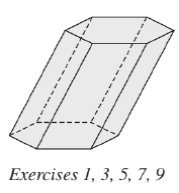Chapter 9.1, Problem 5EElementary Geometry For College St...

7th Edition
Alexander + 2 others
ISBN: 9781337614085

Solutions

Chapter
SectionElementary Geometry For College St...

7th Edition
Alexander + 2 others
ISBN: 9781337614085
Textbook Problem

If each edge of the hexagonal prism in Exercise 1 is measured in centimeters, what unit is used to measure its a ) surface area? b ) volume?To determine

a)

To find:

The unit used to measure lateral area of the hexagonal prism when each of the edge is measured in centimeters.

Explanation

Calculation:

Given,

The lateral area of the hexagonal prism with base perimeter ‘P’ and altitude ‘h’ is given by

L=

To determine

b)

To find:

The unit used to measure volume of the hexagonal prism when each of the edge is measured in centimeters.

Still sussing out bartleby?

Check out a sample textbook solution.

See a sample solution

The Solution to Your Study Problems

Bartleby provides explanations to thousands of textbook problems written by our experts, many with advanced degrees!

Get Started

Find more solutions based on key concepts